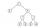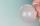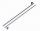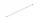# Circles

For the circle c1(S1; r1=146 cm) and c2(S2; r2 = 144 cm) is distance of centers |S1S2| = 295 cm. Determine the distance between the circles.

Result

d =  5 cm

#### Solution:Leave us a comment of example and its solution (i.e. if it is still somewhat unclear...):

Showing 0 comments:Be the first to comment!## Next similar examples:

1. Lake or pondThe landlord has a square lake. Trees grow around this lake. The lake wants to enlarge the pond twice and does not want to cut down or flood any tree. How will he do that?
2. CagesHonza had three cages (black, silver, gold) and three animals (guinea pig, rat and puppy). There was one animal in each cage. The golden cage stood to the left of the black cage. The silver cage stood on the right of the guinea pig cage. The rat was in the
3. Addition of Roman numbersAdded together and write as decimal number: LXVII + MLXIV
4. Eq1Solve equation: 4(a-3)=3(2a-5)
5. ExpressionsFind out value of expressions if a = -1, b =2: x=b - 2a - ab y=a3 - b2 - 2ab z=a2 b3 - a3 b2 w=a + b + a3 - b2
6. If-then equationIf 5x - 17 = -x + 7, then x =
7. CakesOn the bowl were a few cakes. Jane ate one-third of them, Dana ate a quarter of those cakes that remained. a) What part (of the original number of cakes) Dana ate? b) At least how many cakes could be (initially) on thebowl?
8. Flood waterFlood waters in some US village meant that the homes had to evacuate 364 people. 50 of them stayed at elementary schools, 59 them slept with their friends and others went to relatives. How many people have gone to relatives?
9. Foot in busIt was 102 people on the bus. 28 girls had two dogs. A 11 girls had one dog. At the next stop seceded 5 dogs (even with their owners). They got two boys together with three dogs. The bus drove one driver. How many foot were in bus?
10. Ten pupils10 pupils went to the store. 6 pupils bought lollipops and 9 pupils bought chewing gum. How many pupils have bought both lollipops and chewing gums (if everyone bought something)?
11. The resultHow many times I decrease the number 1632 to get the result 24?
12. PlaneOn how many parts divide plane 6 parallels?
13. One frame5 picture frames cost € 12 more than three frames. How much cost one frame?
14. Math classificationIn 3A class are 27 students. One-third got a B in math and the rest got A. How many students received a B in math?
15. Valid numberRound the 453874528 on 2 significant numbers.
16. LineHow many parts of line divide 5 (different) points that lie on it?
17. ZdeněkZdeněk picked up 15 l of water from a 100-liter full-water barrel. Write a fraction of what part of Zdeněk's water he picked.